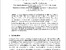# On (4,2)-digraph Containing a Cycle of Length 2

Iswadi, Hazrul and Baskoro, Edy Tri (2000) On (4,2)-digraph Containing a Cycle of Length 2. Bulletin of the Malaysian Mathematical Sciences Society, 23. pp. 79-91. ISSN 01266705Preview PDF hazrul_On (4,2)-digraph Containing a Cycle of Length 2_2000.pdf - Published Version Download (584kB) | Preview

## Abstract

A diregular digraph is a digraph with the in-degree and out-degree of all vertices is constant. The Moore bound for a diregular digraph of degree d and diameter k is M_{d,k}=l+d+d^2+...+d^k. It is well known that diregular digraphs of order M_{d,k}, degree d>l tnd diameter k>l do not exist . A (d,k) -digraph is a diregular digraph of degree d>1, diameter k>1, and number of vertices one less than the Moore bound. For degrees d=2 and 3,it has been shown that for diameter k >= 3 there are no such (d,k)-digraphs. However for diameter 2, it is known that (d,2)-digraphs do exist for any degree d. The line digraph of K_{d+1} is one example of such (42)-digraphs. Furthermore, the recent study showed that there are three non-isomorphic(2,2)-digraphs and exactly one non-isomorphic (3,2)-digraph. In this paper, we shall study (4,2)-digraphs. We show that if (4,2)-digraph G contains a cycle of length 2 then G must be the line digraph of a complete digraph K_5.

Item Type: Article Q Science > QA Mathematics Academic Department > Department of Mathematics and Natural Science Hazrul Iswadi 6179 28 Mar 2012 03:10 24 Mar 2021 14:17 http://repository.ubaya.ac.id/id/eprint/265View Item